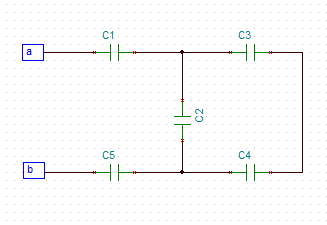# In the figure C_1 = C_5 = 8.3 micro F and C_2 = C_3 = C_4 = 4.4 micro F . The applied potential...

## Question:

In the figure {eq}C_1 = C_5 = 8.3 \ \mu F \ and \ C_2 = C_3 = C_4 = 4.4 \ \mu F {/eq}. The applied potential is {eq}V_{ab} = 210 \ V {/eq}.
What is the equivalent capacitance of the network between points {eq}a \ and \ b?{/eq}
What is the charge and potential difference of each of the capacitors?## Series and Parallel Combination of Capacitors

Many times we require a capacitor whose capacity is not of standard capacity. In order to have such a capacitor, we use a series or parallel combination of capacitors. If we want to have a have a higher capacity than the available capacitors, then the capacitors are connected in parallel. . If we want to have a have a smaller capacity than the available capacitors, then the capacitors are connected in series.

Symbols Used

1) {eq}\space C_1, \space C_2, \space C_3, \space C_4, \space C_5, \space{/eq} are the capacitors

2){eq}\space C_{S1}, \space...

Become a Study.com member to unlock this answer! Create your account